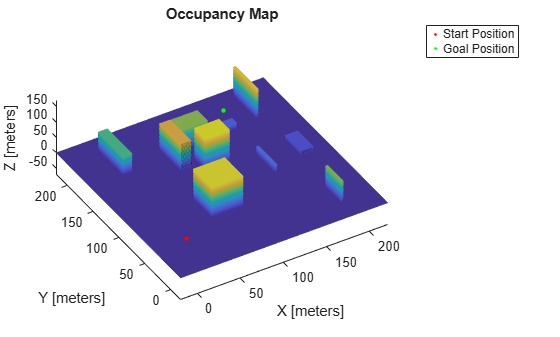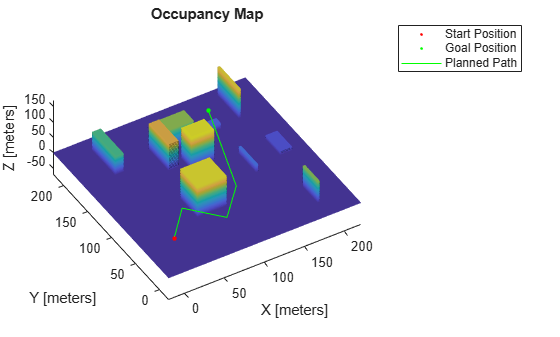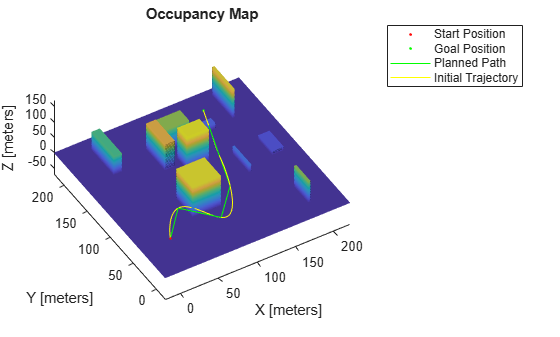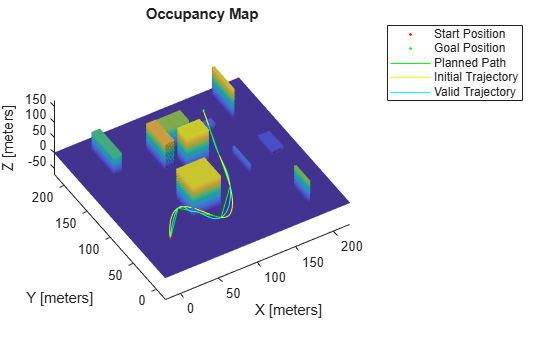# Plan Minimum Snap Trajectory for Quadrotor

This example shows how to plan a minimum snap trajectory (minimum control effort) for a multirotor unmanned aerial vehicle (UAV) from a start pose to a goal pose on a 3D map by using the optimized rapidly exploring random tree (RRT*) path planner.

In this example, you set up a 3D map, provide a start pose and goal pose, plan a path with RRT* using 3D straight line connections, and fit a minimum snap trajectory through the obtained waypoints.

### Initial Setup

Configure the random number generator for repeatable result.

`rng(100,"twister")`

Load the 3D occupancy map `uavMapCityBlock.mat`, which contains a set of pregenerated obstacles, into the workspace. The occupancy map is in an east-north-up (ENU) frame.

```mapData = load("uavMapCityBlock.mat"); omap = mapData.omap; % Consider unknown spaces to be unoccupied omap.FreeThreshold = omap.OccupiedThreshold; show(omap)```

#### Set Start Pose and Goal Pose

Using the map for reference, select an unoccupied start pose and goal pose for the trajectory.

```startPose = [12 22 25 0.7 0.2 0 0.1]; % [x y z qw qx qy qz] goalPose = [150 180 35 0.3 0 0.1 0.6]; % Plot the start and goal poses hold on scatter3(startPose(1),startPose(2),startPose(3),100,".r") scatter3(goalPose(1),goalPose(2),goalPose(3),100,".g") view([-31 63]) legend("","Start Position","Goal Position") hold off```### Plan Path with RRT* Using SE(3) State Space

RRT* is a tree-based motion planner that builds a search tree incrementally from random samples of a given state space. The tree eventually spans the search space and connects the start state and the goal state. Connect the two states with straight line connections using a `stateSpaceSE3` object. Use the `validatorOccupancyMap3D` object for collision checking between the multirotor UAV and the environment.

#### Define State Space Object

The `stateSpaceSE3` object defines the state space as `[x y z qw qx qy qz]`, where `[x y z]` specifies the position of the UAV in meters and `[qw qx qy qz]` specifies the orientation as a quaternion. Specify the position and orientation boundaries of the quadrotor as a 7-by-2 matrix. The orientation boundaries are optional, and can be set to `-Inf` and `Inf` if they are not applicable.

```ss = stateSpaceSE3([-20 220; -20 220; -10 100; inf inf; inf inf; inf inf; inf inf]);```

#### Define State Validator Object

The `validatorOccupancyMap3D` object determines that a state is invalid if the `xyz`-location is occupied on the map. A motion between two states is valid only if all intermediate states are valid, which means the UAV does not pass through any occupied locations on the map. Create a `validatorOccupancyMap3D` object by specifying the state space object and the inflated map. Then set the validation distance, in meters, for interpolating between states.

```sv = validatorOccupancyMap3D(ss,Map=omap); sv.ValidationDistance = 0.1;```

#### Set Up RRT* Path Planner

Create a `plannerRRTStar` object by specifying the state space and state validator as inputs. Set the `MaxConnectionDistance`, `GoalBias`, `MaxIterations`, `ContinueAfterGoalReached`, and `MaxNumTreeNodes` properties of the planner object to optimize the returned waypoints.

```planner = plannerRRTStar(ss,sv); planner.MaxConnectionDistance = 50; planner.GoalBias = 0.8; planner.MaxIterations = 1000; planner.ContinueAfterGoalReached = true; planner.MaxNumTreeNodes = 10000;```

#### Execute Path Planning

Perform RRT* based path planning in 3D space to obtain waypoints. The planner finds a flight path that is collision-free and suitable for the quadrotor. The solution info is helpful for tuning the planner.

`[pthObj,solnInfo] = plan(planner,startPose,goalPose);`

#### Visualize Path

Check if the RRT* planner has found a path. If the planner has found a path, plot the waypoints. Otherwise, terminate the script.

```if (~solnInfo.IsPathFound) disp("No Path Found by the RRT, terminating example") return end % Plot map, start pose, and goal pose show(omap) hold on scatter3(startPose(1),startPose(2),startPose(3),100,".r") scatter3(goalPose(1),goalPose(2),goalPose(3),100,".g") % Plot path computed by path planner plot3(pthObj.States(:,1),pthObj.States(:,2),pthObj.States(:,3),"-g") view([-31 63]) legend("","Start Position","Goal Position","Planned Path") hold off```### Generate Minimum Snap UAV Trajectory

The original planned path has some sharp corners while navigating toward the goal. Generate a smooth trajectory by fitting the obtained waypoints to the minimum snap trajectory using the `minsnappolytraj` function. For your initial estimate of the time required to reach each waypoint, assume the UAV moves at a constant speed.

To tune the trajectory and flight time, specify the `numSamples`, `TimeAllocation`, and `TimeWeight` arguments of the `minsnappolytraj` function.

```waypoints = pthObj.States; nWayPoints = pthObj.NumStates; % Calculate the distance between waypoints distance = zeros(1,nWayPoints); for i = 2:nWayPoints distance(i) = norm(waypoints(i,1:3) - waypoints(i-1,1:3)); end % Assume a UAV speed of 3 m/s and calculate time taken to reach each waypoint UAVspeed = 3; timepoints = cumsum(distance/UAVspeed); nSamples = 100; % Compute states along the trajectory initialStates = minsnappolytraj(waypoints',timepoints,nSamples,MinSegmentTime=4,MaxSegmentTime=20,TimeAllocation=true,TimeWeight=100)';```

#### Visualize Trajectory

Visualize the obtained minimum snap trajectory.

```% Plot map, start pose, and goal pose show(omap) hold on scatter3(startPose(1),startPose(2),startPose(3),30,".r") scatter3(goalPose(1),goalPose(2),goalPose(3),30,".g") % Plot the waypoints plot3(pthObj.States(:,1),pthObj.States(:,2),pthObj.States(:,3),"-g") % Plot the minimum snap trajectory plot3(initialStates(:,1),initialStates(:,2),initialStates(:,3),"-y") view([-31 63]) legend("","Start Position","Goal Position","Planned Path","Initial Trajectory") hold off```#### Generate Valid Minimum Snap Trajectory

Notice that the generated flight trajectory has some invalid states, which are not obstacle-free. You must modify the waypoints so that the generated trajectory is obstacle-free. To avoid invalid states, add intermediate waypoints on the segment between the pair of waypoints for which the trajectory is invalid. Insert waypoints iteratively until the entire trajectory is valid.

```% Check if the trajectory is valid states = initialStates; valid = all(isStateValid(sv,states)); while(~valid) % Check the validity of the states validity = isStateValid(sv,states); % Map the states to the corresponding waypoint segments segmentIndices = exampleHelperMapStatesToPathSegments(waypoints,states); % Get the segments for the invalid states % Use unique, because multiple states in the same segment might be invalid invalidSegments = unique(segmentIndices(~validity)); % Add intermediate waypoints on the invalid segments for i = 1:size(invalidSegments) segment = invalidSegments(i); % Take the midpoint of the position to get the intermediate position midpoint(1:3) = (waypoints(segment,1:3) + waypoints(segment+1,1:3))/2; % Spherically interpolate the quaternions to get the intermediate quaternion midpoint(4:7) = slerp(quaternion(waypoints(segment,4:7)),quaternion(waypoints(segment+1,4:7)),.5).compact; waypoints = [waypoints(1:segment,:); midpoint; waypoints(segment+1:end,:)]; end nWayPoints = size(waypoints,1); distance = zeros(1,nWayPoints); for i = 2:nWayPoints distance(i) = norm(waypoints(i,1:3) - waypoints(i-1,1:3)); end % Calculate the time taken to reach each waypoint timepoints = cumsum(distance/UAVspeed); nSamples = 100; states = minsnappolytraj(waypoints',timepoints,nSamples,MinSegmentTime=2,MaxSegmentTime=20,TimeAllocation=true,TimeWeight=5000)'; % Check if the new trajectory is valid valid = all(isStateValid(sv,states)); end```

#### Visualize Final Valid Trajectory

Visualize the final valid minimum snap trajectory.

```% Plot map, start and goal pose show(omap) hold on scatter3(startPose(1),startPose(2),startPose(3),30,".r") scatter3(goalPose(1),goalPose(2),goalPose(3),30,".g") % Plot the waypoints plot3(pthObj.States(:,1),pthObj.States(:,2),pthObj.States(:,3),"-g") % Plot the initial trajectory plot3(initialStates(:,1),initialStates(:,2),initialStates(:,3),"-y") % Plot the final valid trajectory plot3(states(:,1),states(:,2),states(:,3),"-c") view([-31 63]) legend("","Start Position","Goal Position","Planned Path","Initial Trajectory","Valid Trajectory") hold off```